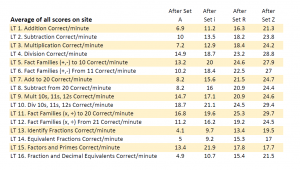## A Data-Proven Approach to Measuring Math Facts Fluency (That Kids Love!)

You know your student is building math facts fluency, but can you quantify it? With Rocket Math’s Online Game, you can track your student’s progress learning addition, subtraction, multiplication, and division (and 12 other Learning Tracks) from level A to Z. Progress is tracked numerically through 1-Minute Races so that you can compare results over time, between students, and across classrooms.

## How Rocket Math’s 1-Minute Races Measure Math Fact Fluency

Starting in the Fall of 2020, the Rocket Math Online Game began scheduling 1-Minute Races for students as they worked through the 16 different Learning Tracks taught in the game.

The 1-Minute Races present a random selection of all the facts in each Learning Track. Students are encouraged to skip facts they haven’t learned, but the scores shown here are the correct answers. When a student completes sets A, i, R, and Z, the 1-Minute Races (also known as math facts fluency tests) are scheduled. We wait until after students complete set A to give the first pre-test, so we know the students know how to use the interface and answer questions.

As the chart below shows, Set A represents the average student’s starting score. Set i and R show intermediate progress. And finally, Set Z represents the average fluency students have achieved by playing through levels 26 A to Z in that Learning Track.

#### Average 1-Minute Race Scores of Students Playing the Rocket Math Online GameThe more correct problems per minute, the more fluent the learner. On average, by the end of a Learning Track students should aim for 20 to 30 correct problems per minute in the Online Game races. Want to learn more about how fast students should be with math facts? Read here.

## Data show improved math facts fluency as students work through each Learning Track

As you can see above, there is a steady rise in fluency as students work through each Learning Track. Because fluency scores go up consistently at each measuring point, and they do so in every Learning Track, we can be sure that the Online Game is effectively developing fluency.

## Data shows improvement based on how many math facts answered per minute

The fact that on each teststudents can correctly answer more problems shows that they are learning, and the data proves it. That is very important, as you don’t purchase this game simply to entertain children. The goal is to help students achieve math facts fluency, and we are seeing that here. Individual results will, of course, vary.

## Not only an excellent online math speed test but also an excellent tool for research

Teachers can also assign these 1-Minute Races at any time, or even on a weekly basis. The scheduled tests, however, show the best effect of the Online Game because the amount of work done to achieve the tests is equal among students. Both scheduled and teacher-assigned results are kept, and the results of these tests can be exported in excel files, so they are available for research purposes. We invite you to study your students’ math fact fluency using the Rocket Math Online Game and 1-Minute Races. One thing is certain from our data, the Rocket Math Online Game works!